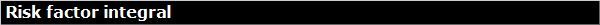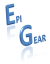Home Ersatz MetaXL DisMod II EpigearXL OR2RR Risk factor Publications Contact Obituary AboutRisk factor IntegralA comparison of three different methods to calculate the potential impact fraction of an intervention. See the example spreadsheet in the download for details. Description An important measure of effect on the population level, used to calculate the impact on risk for disease, given a risk factor intervention, is the potential impact fraction (PIF). The PIF is mostly calculated using a categorical risk factor distribution and a relative risk for each category. However, some risk factors, such as hypertension and BMI, are much better described by continuous distributions.When a PIF is calculated from a continuous risk factor distribution, it is necessary to calculate the integral of the product of the risk factor distribution and the corresponding relative risk function (see the paper in the download for details). The risk factor integral add-in provides this functionality for Excel.Several risk factor distributions and risk functions are available. A basic understanding of integration will be helpful to set correct integration boundaries. Features Normal, Lognormal, Weibull, and user-defined risk factor distributionsLinear, Loglinear, Logit, per unit, and user-defined relative risk functions Percentile functions for all risk factor distributionsHigh precision numerical integration method (Romberg integration)Product Summary Risk factor integral is a set of functions that calculate population risk exposure by integrating the product of a continuous risk factor prevalence distribution and a relative risk function. The result can be used to calculate potential impact fractions population attributtable fractions. The functions were first implemented in a stand-alone Excel add-in, but are now subsumed in the EpigearXL add-in.[Home] [Products] [Publications] [Contact] [Obituary] [About EpiGear]© Copyright 2016 EpiGear International Pty Ltd.  All rights reserved.